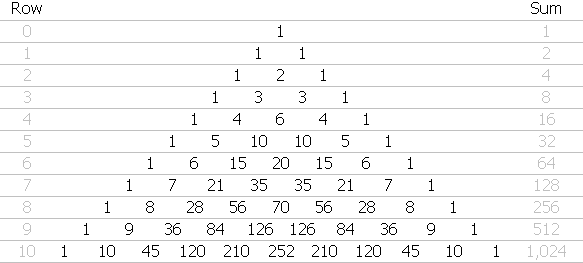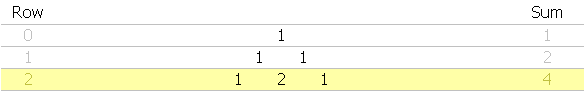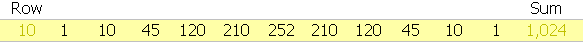# Combinations and Pascal’s Triangle, part 1

Recently I needed to calculate all the possible combinations for these modifier keys:

Alt, Control, Shift, Window (abbreviated as A,C,S,W)

…ranging from none of them to all four, and every combination in between. I didn’t want to miss any of them, so calculating the total number of possible combinations seemed like a good idea. (As you may have noticed, this was on a Windows computer, and no, I didn’t forget Caps Lock — it wasn’t relevant in this case.)As it happens, there is a very simple formula, 2^x, where x represents the total number of objects, for counting all possible combinations (including none).

So, the answer was 2^4, or 16, as follows: A, AC, AS, AW, ACS, ACW, ASW, ACSW, C, CS, CW, CSW, S, SW, W and none.And, of course, if I only wanted to count actual key combos, and didn’t care about the “no keys” result, then the correct formula would instead be the one shown at the right.All this put me in mind of Pascal’s Triangle, which can be used to answer this question, as well as many others. On the off-chance you’re not familiar with Pascal’s Triangle, you start with a 1, and place two 1’s below it, offset to either side, like so.

From there, you add as many rows as you wish, according to this recipe: each row begins and ends with a 1; all intervening entries are sum of the two offset numbers above. Here’s a ten row example (it’s actually 11 rows; the first row is considered to be row zero):Notice any correspondence between the row number and the horizontal sum of the digits? (If you don’t imediately see it, take a minute and think about it before reading further.)

If you said, “By golly, each row number corresponds to a power of 2,” give yourself a gold star. So the answer to my original question can be determined by going to row 4 and summing the digits in that row… and sure enough, the answer is 16.

Incidentally, one of my favorite uses for Pascal’s Triangle is answering questions like, “If you flip a coin ten times in a row, what are the odds you’ll get heads five times and tails five times?” If you think the answer is 50/50, Pascal’s Triangle will quickly disabuse you of that notion.

But before we tackle ten tosses, let’s look at a what happens if we toss a coin twice… and for that we go to row 2, which corresponds to the number of tosses. The “Sum” value at the right represents the number of possible outcomes, which are HH, HT, TH, and TT.In plain English we would say, “You’ll either get two heads, one of each, or two tails,” and moving from left to right, the first 1 corresponds to HH, the 2 in the middle represents HT and TH, and the 1 at the right represents TT. So, the odds are 1-in-4 that we’ll get HH, 2-in-4 that we’ll get one of each, and 1-in-4 that we’ll get TT.

Every row in Pascal’s triangle works this way, so now we can answer the earlier coin toss question: If you flip a coin ten times, what are the odds you’ll get five heads and five tails?Working our way from left to right, the first value corresponds to the odds for 10H (all heads), i.e., 1-in-1024. The next value shows the odds for 9H1T (nine heads, one tail), or 10-in-1024, and so on, until we reach the middle position, corresponding to 5H5T, and we see that the odds are 252-in-1024, which works out to 24.6% — hardly the 50% odds that some people would assume.

In part 2 we’ll take a look at a related problem, which can be solved using either a modified version of Pascal’s Triangle, or a function most FileMaker developers never touch, and many have never heard of: the Combination function.

## 2 thoughts on “Combinations and Pascal’s Triangle, part 1”

1. Kaare Bjorvik says:

WRONG! On line 10, it is 120 not 124.

1. Kevin Frank says:

Good catch, Kaare… I have now fixed it in this article and in part 2 as well. Thanks.

This site uses Akismet to reduce spam. Learn how your comment data is processed.